# AFSK Digital Correlator Demodulator¶

This notebook contains a Python implementation of the 1200 baud AFSK demodulator used in the Mobilinkd TNC3.

This started out as a short demo in Python of the demodulator presented in PSoC ® 1 - Simplified FSK Detection (AN2336) by Dennis Seguine for Cypress Semiconductor. It has grown quite a bit since. This now expands on that application note to show a complete HDLC packet demodulator/decoder.

The purpose of this design is to demodulate 1200 baud amateur radio packet data. This encompasses the following topics:

• AFSK Demodulation (the topic of the above mentioned appliction note)
• Clock Recovery
• Data Carrier Detection
• HDLC Decoding
• AX.25 Packet Parsing

## AFSK Basics¶

AFSK -- audio frequency shift keying -- is a method of sending data by alternating two tones, called mark and space, to transmit binary data.

Amateur radio packet data uses the Bell 202 modem specification, which specifies a 1200 baud data rate, 1200Hz mark and 2200Hz space tones, a +/- 10Hz accuracy, and a 1dB flatness for the audio signal. Bell 202 is a phase-continuous modulation scheme, meaning that there are no abrupt phase changes when switching between mark and space tones.

### NRZI¶

The tones themselves do not encode 0/1 directly. Rather a non-return to zero, inverted (NRZI) scheme is used. What this means in laymans terms is that rather than the tones themselves encoding 0s and 1s, it is a change or no change in tone that encodes the data. In NRZI, a change in tone encodes a 0 and no change in tone encodes a 1.

## AFSK Challenges¶

Digital signal processing of AFSK data presents a number of challenges. At the most rudimentary level we have to deal with noise in the signal and have to overcome low SNR. In practice, low SNR is actually the least of our worries. we are focused on AFSK over FM, which provides a fairly low SNR until it hits a cliff and falls off very fast.

A much more serious problem we face is twist. Twist is the amplitude difference between mark and space tones. Recall that the Bell 202 spec requires a 1dB flatness. Over an amateur radio voice channel, at its worst we can have twist of +/-9dB and absolutely must handle twist of 6dB.

1. The voice channel is emphasized on transmission and de-emphasized on reception. Pre-emphasis adds about 6dB to the space tone and then subtracts 6dB during de-emphasis.
2. Not all radios do pre-emphasis. This can cause up to 6dB in twist when received on a radio that does de-emphasis.
3. Radios that do pre-emphasis may clip the signal after emphasis. This causes the higher tone to be lower in amplitude when de-emphasized. This can cause up to 6dB in twist when de-emphasized.
4. Some people may use radios that do not apply de-emphasis. This may present 6dB twist to the modem in the other direction.

Because AFSK is sent over a voice channel, and is sent and received using equipment designed for voice communication, we have to deal with audio processing artifacts that are typically avoided in data transmission. The audio filters, pre-emphasis and de-emphasis filters and limiters used can cause phase shifts in audio that is imperceptible to human ears but which can lead to inter-symbol interference (ISI) when sending data.

At least one major TNC manufacturer substituted V.23 modem chips (rather than Bell 202) in their product for a time, causing tones to by out of spec by 100Hz.

All modern digital communications standards use some form of forward error correction (FEC). 1200 baud packet radio uses no FEC. A one-bit error will prevent a packet from being properly decoded.

So, to summarize, our modem must deal with:

• Low SNR -- noise on top of the actual data signal
• High twist -- large differences in amplitude between mark and space tones
• Phase distortion -- mark and space tones not arriving at symbol boundaries
• Inter-symbol interference -- mark and space tones overlapping due to phase distortion
• Frequency distortion -- large differences in tone frequency and data rate
• No error correction -- one bit errors are fatal

These issues make demodulating a packet radio signal more challenging than 1200 baud over POTS. In order to acheive best in class performance the demodulator needs to address all of these issues.

## AFSK Demodulation¶

As mentioned above, there are a number of steps necessary in order to get to a decoded packet. The first step is demodulation -- turning the tones into bits. This is a digital correlator demodulator. It is very simple to implement. It consumes very little resources which is important when running on low-power emdedded devices. And it is fairly tolerant of twist.

### What is a Digital Correlator¶

The correlator involves little more than multiplying the input signal with a delayed copy of itself. It is a comb filter. It causes the signal to destructively and constructively interfere with itself in such a manner as to demodulate the encoded bitstream. By adjusting the delay, we can maximize the difference between mark and space tones.

A digital correlator invovles converting the analog signal into a digital one by way of a zero-crossing detector, and then applying the same delay as one would with an analog comb filter.

An analog comb filter is sensitive to twist and is more costly to implement than a digital one. A digital correlator is more sensitive to low-frequency noise and simpler to implement in code or digital circuitry.

Note: it may be cheaper to deal with the twist issues with an analog comb filter.

$y[n]=x[n]+\alpha x[n-K]$

$\alpha$ is a scaling factor that involves a single multiply per sample.

### Finding the Right Delay¶

As mentioned above, we are designing a comb filter where the goal is to maximize the difference between mark and space tones. This is a problem summed up by:

$y[d] = cos(2\pi f_L d) - cos(2 \pi f_H d)$

where $f_L$ is the low freqency, $f_H$ is the high frequency and $d$ is the delay.

We can graph this equation. When we do, we should see what delays provides the biggest differences between the two tones.

In :
%matplotlib notebook
import matplotlib.pyplot as plt

def plot_to_notebook(time_sec,mark_signal,space_signal,corr_signal,n_samples):
plt.figure()
plt.subplot(1, 1, 1)
plt.xlabel('Time (msec)')
plt.grid()
plt.plot(time_sec[:n_samples]*1000,mark_signal[:n_samples],'y-',label='Mark signal')
plt.plot(time_sec[:n_samples]*1000,space_signal[:n_samples],'g-',label='Space signal')
plt.plot(time_sec[:n_samples]*1000,corr_signal[:n_samples],'r-',linewidth=2,label='Correlation')
plt.legend()

In :
import numpy as np

# Total time
T = 0.001
# Sampling frequency
fs = 1e6
# Number of samples
n = int(T * fs)
# Time vector in seconds
t = np.linspace(0, T, n, endpoint=False)
# Samples of the signals
mark = np.cos(1200*2*np.pi*t)
space = np.cos(2200*2*np.pi*t)
corr =  np.cos(2200*2*np.pi*t) - np.cos(1200*2*np.pi*t)
# Convert samples to 32-bit integers
# samples = samples.astype(np.int32)
print('Number of samples: ',n)

# Plot signal to the notebook
plot_to_notebook(t,mark,space,corr,1000)

Number of samples:  1000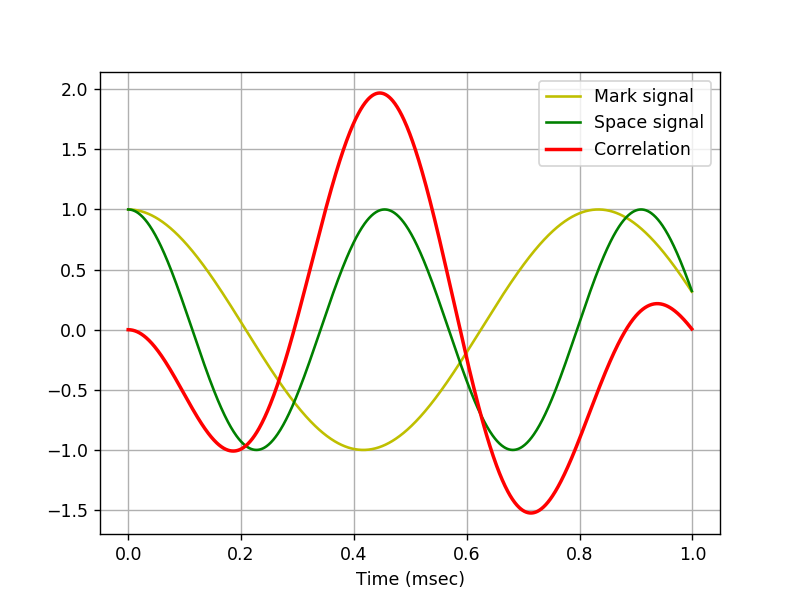Here we see that a time of 446us will lead to a difference of 2. This matches the value provided in the Cypress application note.

As mentioned above, some of the modems found in the wild on APRS actually use the V.23 standard (1300/2100Hz tones).

Let's let's see what impact that will have on our correlator.

In :
import numpy as np

# Total time
T = 0.001
# Sampling frequency
fs = 1e6
# Number of samples
n = int(T * fs)
# Time vector in seconds
t = np.linspace(0, T, n, endpoint=False)
# Samples of the signals
mark = np.cos(1300*2*np.pi*t)
space = np.cos(2100*2*np.pi*t)
corr =  np.cos(2100*2*np.pi*t) - np.cos(1300*2*np.pi*t)
# Convert samples to 32-bit integers
# samples = samples.astype(np.int32)
print('Number of samples: ',n)

# Plot signal to the notebook
plot_to_notebook(t,mark,space,corr,1000)

Number of samples:  1000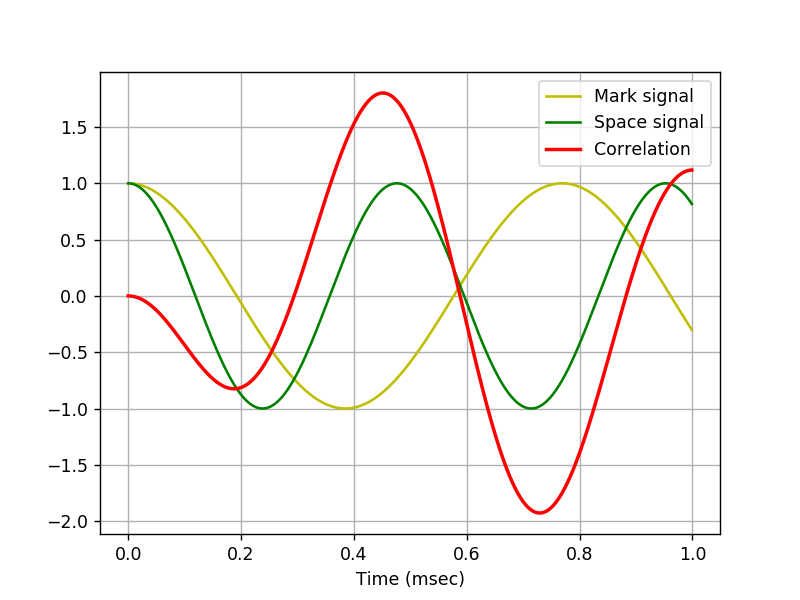Notice that the maximum is at 730us. However, the first correlation maximum corresponds nicely with the maximum for the Bell 202 standard, with a value of 1.8. According to the Cypress application note, this should be good enough.

We can try some empircal tests later to determine if using a 730us delay has a meaningful impact.

Towards the end of the Cypress application note, the author states that the sample rate should be an integer multiple of the two tones. It is impossible to find a reasonable sample rate that meets this criterion for both Bell 202 and V.23 since the two standards have integer multiples of 2, 3, 7, 11 and 13 (it would be 6,006,000 samples per second).

This difference in sample rate may be more significant than the correlator delay.

## Implementation¶

This section will start the implementation of the correlator. To start, we will load a 1 second audio file from a WA8LMF APRS packet test track. This is from 10 seconds into track 2. This is chosen because this one-second snippet is known to have data at the start, noise in the middle, and a complete packet towards the end.

The sample file was generated using the following command:

\$ sox TNC_Test_Ver-1.102.wav TNC_Test_Ver-1.102-26400-1sec.wav trim 10 1.05 rate 26400 channels 1



We grabbed a little more than one full second in order to provide the additional data needed to for the correlator and to accommodate our block sampling code at the end. We chose a 26400 sample rate because it is an even multiple of our data rate and two tones.

We will use scipy to read the WAV file. The audio file is read into a tuple containing the sample rate and a numpy array of audio data. Because this is a single channel sample, the array is 1-dimensional.

In :
from scipy.io.wavfile import read

print(audio_file)

(26400, array([719, 748, 468, ..., 864, 797, 582], dtype=int16))


### Audio Visualization¶

Lets look at what the audio signal looks like. The audio picks up in the middle of a packet transmission, so we should see live data.

Let's look at the first few bits.

In :
sample_rate = audio_file
audio_data = audio_file

def plot_audio(time_sec,n_samples,signal,filtered=None):
plt.figure()
plt.subplot(1, 1, 1)
plt.xlabel('Time (msec)')
plt.grid()
plt.plot(time_sec[:n_samples]*1000,signal[:n_samples],'y-',label='Audio signal')
if filtered is not None:
plt.plot(time_sec[:n_samples]*1000,filtered[:n_samples],'g-',label='Filtered signal')
plt.legend()

# Total time
T = 10.0 / 1200.0
# Sampling frequency
fs = sample_rate
# Number of samples
n = int(T * fs)
# Time vector in seconds
t = np.linspace(0, T, n, endpoint=False)

print('Number of samples: ',n)

# Plot signal to the notebook
plot_audio(t,n,audio_data[140:])

Number of samples:  220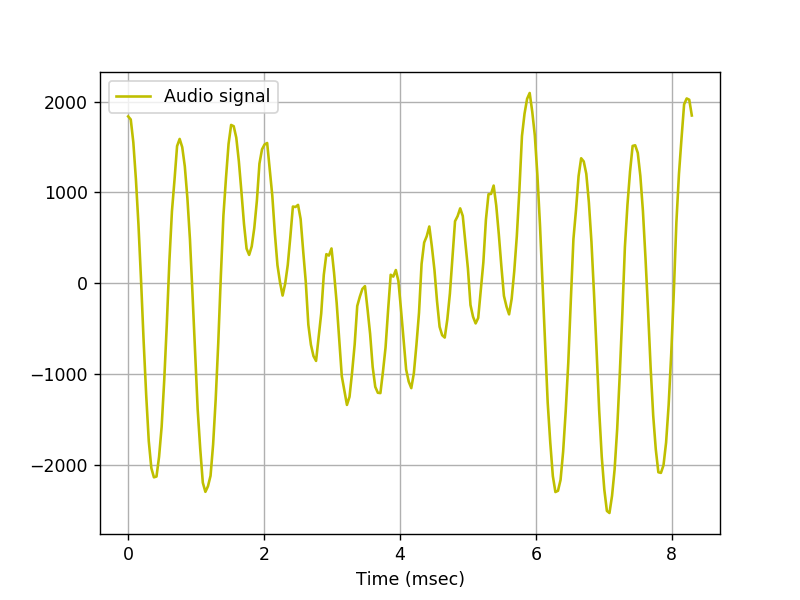There is certainly data here. We can see the distinct differences between the 1200Hz and 2200Hz mark and space tones. We can also see an example of twist here. The 2200Hz tone has a significantly lower amplitude than the 1200Hz tone. This is a rather noisy signal. There is major low-frequency component that would make digitizing this signal with a zero-crossing detector problematic.

Before this signal can be demodulated it must be filtered.

To do this, we construct a band-pass filter using scipy.filter. We are going to use a rather steep FIR filter with cut-offs very close to our band of interest (1200-2200Hz).

A FIR filter is used for its linear phase response. We don't want to add to the ISI with our own digital processing.

In :
from scipy.signal import lfiltic, lfilter, firwin

class fir_filter(object):
def __init__(self, coeffs):
self.coeffs = coeffs
self.zl = lfiltic(self.coeffs, 32768.0, [], [])
def __call__(self, data):
result, self.zl = lfilter(self.coeffs, 32768.0, data, -1, self.zl)
return result

bpf_coeffs = np.array(firwin(141, [1100.0/(sample_rate/2), 2300.0/(sample_rate/2)], width = None,
pass_zero = False, scale = True, window='hann') * 32768, dtype=int)

print(bpf_coeffs)

bpf = fir_filter(bpf_coeffs)

filter_delay = len(bpf_coeffs)//2

delay = 12

samples = n+filter_delay+delay
audio_samples = audio_data[140:140+samples]
filtered_audio = bpf(audio_samples)[filter_delay:]
plot_audio(t,n,audio_samples,filtered_audio)

[    0     0     0     0     0     0     1     3     5     8     8     5
-2   -13   -27   -40   -46   -44   -32   -12    11    32    44    44
32    14     0    -2    13    49    97   143   170   160   104     6
-118  -244  -340  -381  -352  -258  -120    24   138   192   173    97
0   -67   -56    62   287   575   850  1021  1001   737   228  -462
-1216 -1879 -2293 -2336 -1956 -1182  -133  1008  2030  2736  2988  2736
2030  1008  -133 -1182 -1956 -2336 -2293 -1879 -1216  -462   228   737
1001  1021   850   575   287    62   -56   -67     0    97   173   192
138    24  -120  -258  -352  -381  -340  -244  -118     6   104   160
170   143    97    49    13    -2     0    14    32    44    44    32
11   -12   -32   -44   -46   -40   -27   -13    -2     5     8     8
5     3     1     0     0     0     0     0     0]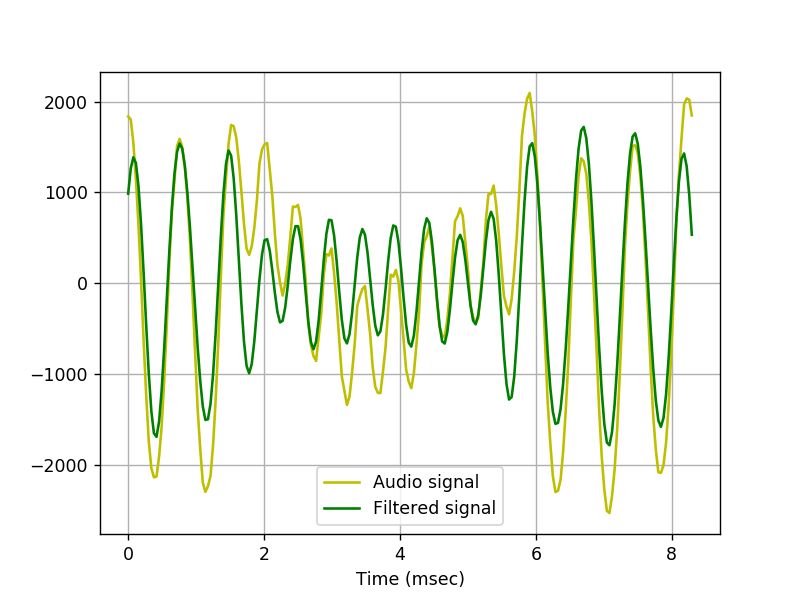Note that the low-frequency component has been mostly eliminated, allowing a zero-crossing digitizer to work properly. The importance of good band-pass filtering of the input audio signal cannot be overstated.

## Digitizing the Audio Signal¶

Now lets look at what happens when we digitize the audio signal.

In :
digitized = np.array([int(x > 0) for x in filtered_audio])

fig, ax = plt.subplots(2, sharex=True)
plt.xlabel('Time (msec)')
plt.title("Digitized")

ax.plot(t[:n]*1000,filtered_audio[:n],'y-')
ax.set_ylabel("Filtered")
ax.grid()
ax.plot(t[:n]*1000,digitized[:n],'g-')
ax.set_ylabel("Digitized")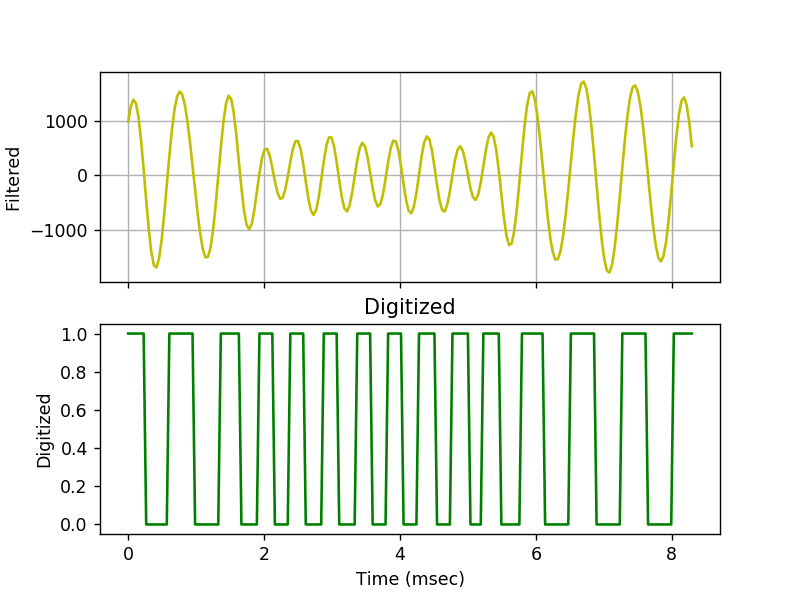Out:
Text(0,0.5,'Digitized')

The mark and space tones, visible in the analog input, are also apparent in the digitized form. The twist in the analog signal is no longer a problem.

Heavy twist can still cause problems for this digital correlator, so it should not be dismissed as a solved problem here. But it is a less significant problem in this modulator than with other demodulator architectures.

### Comb Filter¶

Recall that a correlator is a comb filter -- a signal that is multiplied by a delayed version of itself. In the binary world, XOR will accomplish the multiplication for us.

Lets take a look at the digital version, it's delayed version, and the correlator output.

In :
delayed = digitized[delay:]
xored = np.logical_xor(digitized[:0-delay], delayed)

fig, ax = plt.subplots(3, sharex=True)
plt.xlabel('Time (msec)')
plt.title("Digitized")
plt.grid()
ax.plot(t[:n]*1000,digitized[:n],'y-')
ax.set_ylabel("Digitized")
ax.plot(t[:n]*1000,delayed[:n],'g-')
ax.set_ylabel("Delayed")
ax.plot(t[:n]*1000,xored[:n],'r-')
ax.set_ylabel("XORed")